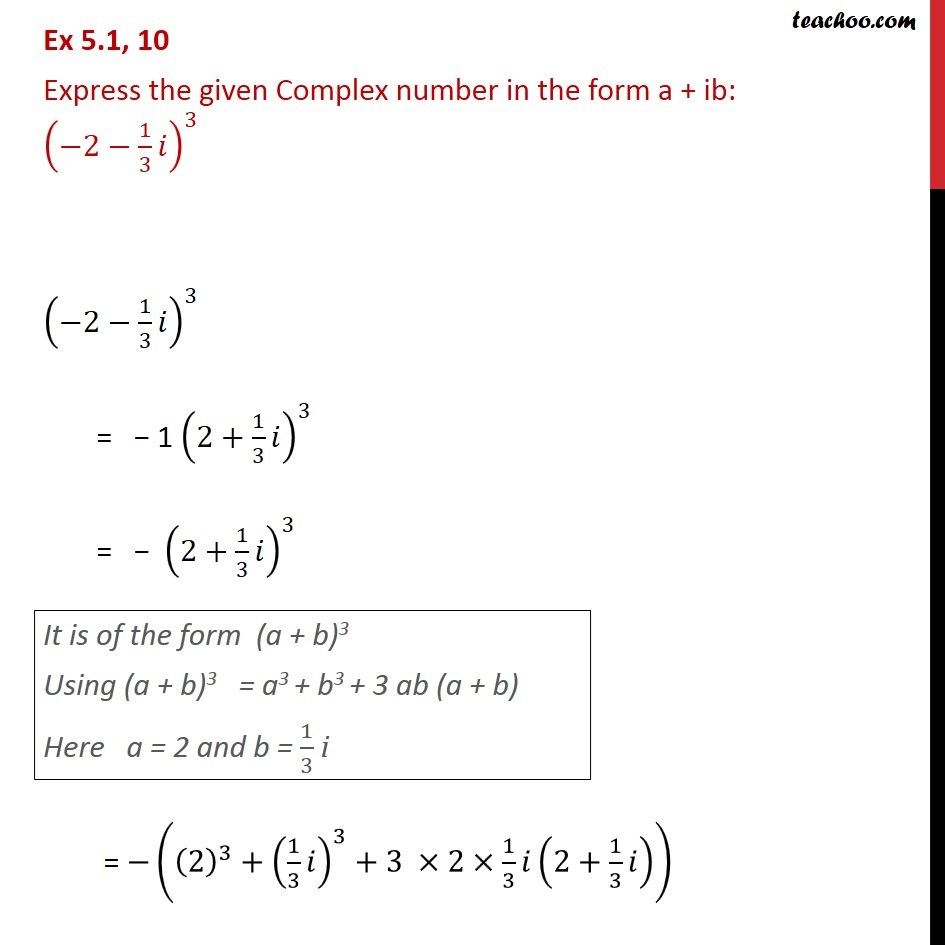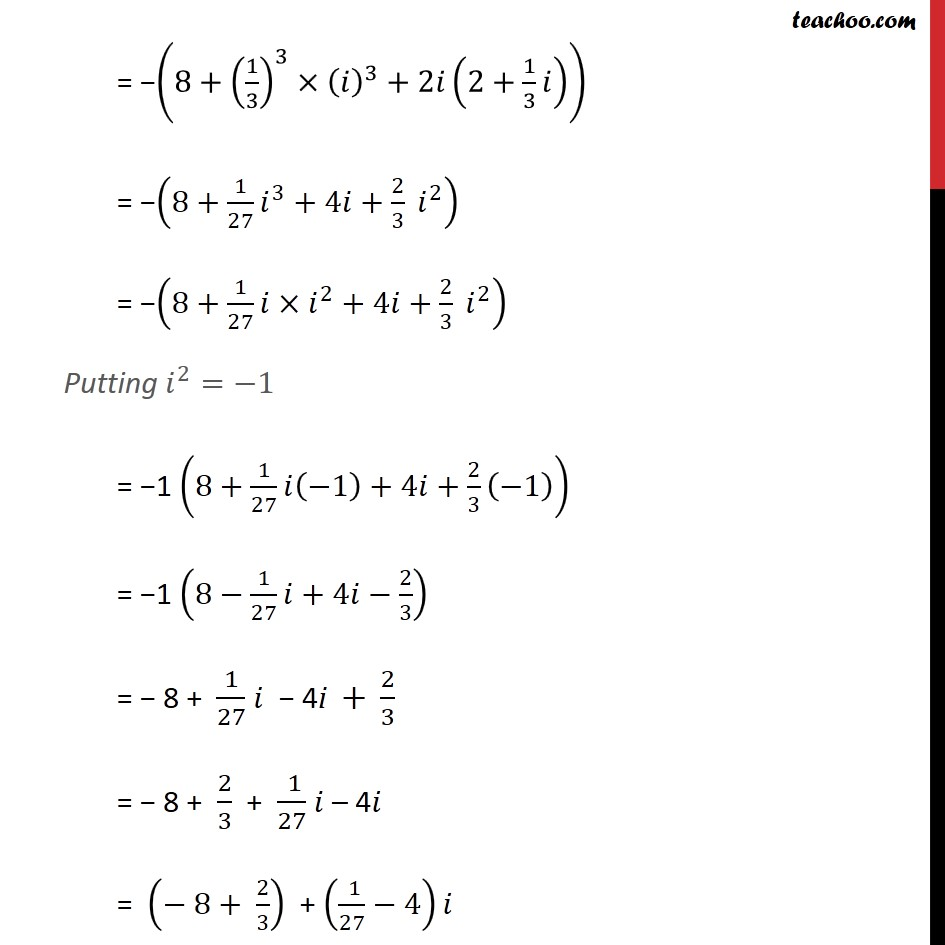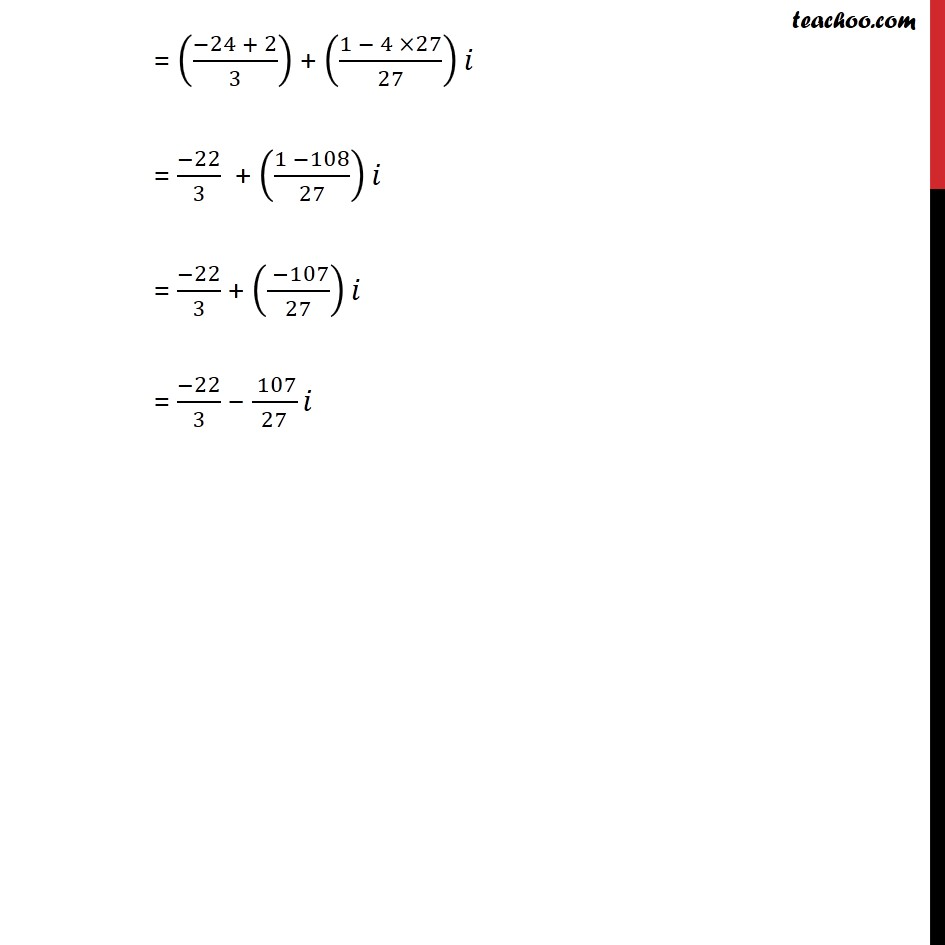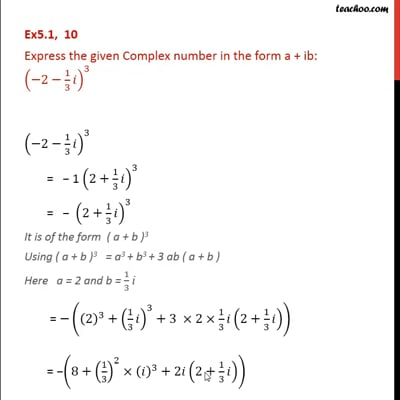Identities (square, cube of 2 complex numbers)

Chapter 5 Class 11 Complex Numbers
Concept wiseThis video is only available for Teachoo black users

Introducing your new favourite teacher - Teachoo Black, at only ₹83 per month

### Transcript

Ex 5.1, 10 Express the given Complex number in the form a + ib: (−2−1/3 𝑖)^3 (−2−1/3 𝑖)^3 = − 1 (2+1/3 𝑖)^3 = − (2+1/3 𝑖)^3 It is of the form (a + b)3 Using (a + b)3 = a3 + b3 + 3 ab (a + b) Here a = 2 and b = 1/3 i = −((2)^3+(1/3 𝑖)^3+3 ×2×1/3 𝑖(2+1/3 𝑖)) = −(8+(1/3)^3×(𝑖)^3+2𝑖(2+1/3 𝑖)) = −(8+1/27 𝑖^3+4𝑖+2/3 𝑖^2 ) = −(8+1/27 〖𝑖×𝑖〗^2+4𝑖+2/3 𝑖^2 ) Putting 𝑖^2=−1 = −1 (8+1/27 𝑖(−1)+4𝑖+2/3 (−1)) = −1 (8−1/27 𝑖+4𝑖−2/3) = − 8 + 1/27 𝑖 − 4i + 2/3 = − 8 + 2/3 + ( 1)/27 𝑖 – 4𝑖 = (− 8+ 2/3) + (( 1)/27−4)𝑖 = ((−24 + 2)/3) + ((1 − 4 ×27)/27)𝑖 = (−22)/3 + ((1 −108)/27)𝑖 = (−22)/3 + (( −107)/27)𝑖 = (−22)/3 − ( 107)/27 𝑖